Courses

# RD Sharma Solutions (Part - 3) - Ex-14.2, Lines and Angles, Class 7, Math Class 7 Notes | EduRev

## Class 7: RD Sharma Solutions (Part - 3) - Ex-14.2, Lines and Angles, Class 7, Math Class 7 Notes | EduRev

The document RD Sharma Solutions (Part - 3) - Ex-14.2, Lines and Angles, Class 7, Math Class 7 Notes | EduRev is a part of the Class 7 Course RD Sharma Solutions for Class 7 Mathematics.
All you need of Class 7 at this link: Class 7

#### Question 28:

In Fig., AB || CD and AC || BD. Find the values of xyz.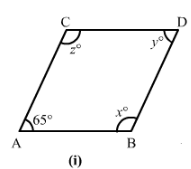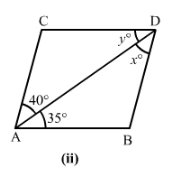(i) Since AC || BD and CD || AB, ABCD is a parallelogram.
∠CAB + ∠ACD = 180°     (Sum of adjacent angles of a parallelogram)
∴ ∠ACD = 180° − 65° = 115°
∠CAD = ∠CDB = 65°         (Opposite angles of a parallelogram)
∠ACD = ∠DBA = 115°       (Opposite angles of a parallelogram)

(ii) Here,
AC || BD and CD || AB
∠DAC = x = 40°            (Alternate interior angle)
∠DAB = y = 35°            (Alternate interior angle)

#### Question 29:

In Fig., state which lines are parallel and why?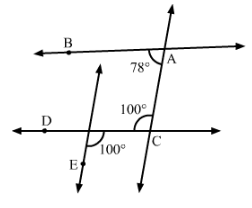Since ∠ACD and ∠CDE are alternate and equal angles, so
∠ACD = 100° = ∠CDE
∴ AC || EF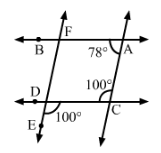#### Question 30:

In Fig. 87, the corresponding arms of ∠ABC and ∠DEF are parallel. If ∠ABC = 75°, find ∠DEF.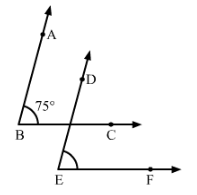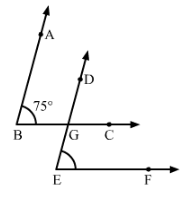Construction : Let G be the point of intersection of lines BC and DE.

∵ AB || DE and BC || EF

∴ ∠ABC=∠DGC=∠DEF=75°∠ABC=∠DGC=∠DEF=75°  (Corresponding angles)

The document RD Sharma Solutions (Part - 3) - Ex-14.2, Lines and Angles, Class 7, Math Class 7 Notes | EduRev is a part of the Class 7 Course RD Sharma Solutions for Class 7 Mathematics.
All you need of Class 7 at this link: Class 7Use Code STAYHOME200 and get INR 200 additional OFF Use Coupon Code
All Tests, Videos & Notes of Class 7: Class 7

### Top Courses for Class 7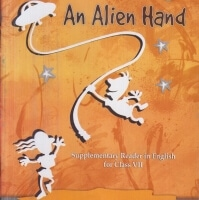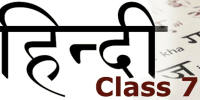## RD Sharma Solutions for Class 7 Mathematics

97 docs

### Top Courses for Class 7Track your progress, build streaks, highlight & save important lessons and more!

,

,

,

,

,

,

,

,

,

,

,

,

,

,

,

,

,

,

,

,

,

,

,

,

,

,

,

,

,

,

;